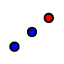# Triangle Around a Centroid

## What is the Construction?

In this construction, I begin with a point that is the centroid of SOME triangle. Knowing that the centroid divides the median in a 2:1 ratio, and that one end of the median is the midpoint of one of the sides of the triangle, I repeatedly use theReflect in a Point tool to find the points needed in the construction.

## What does Wikipedia say about the Centroid?

Is the Centroid your favorite triangle center?

Select all that apply
• A
• B
• C